# Fundamental Data Types and Conversions

Tom Kelliher, CS18

Mar. 1, 1996

# Fundamental Data Types

## Integer Types

char, int

Attributes:

• signed/ unsigned (does not affect memory allocated)
• int is signed by default
• Default for char is implementation dependent
• short/ long (applicable to int only)

Note:

• char can store an integer
• int can store an ASCII value
• Strings must be stored in `char[]`

Memory allocation: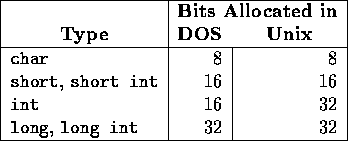### Representation Ranges (Repeat)

Signed, unsigned two's complement representation

Consider the eight bit number:Signed value: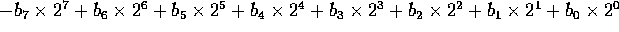Unsigned value: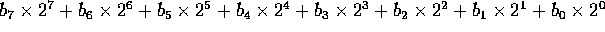Examples:

• 01010101
• 11111001
• 01101001
• 10000101

Smallest, largest values for each representation?

How many unique values can be represented by n bits?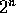, proven by induction on n

Basis: n = 1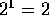and a one bit number has two unique values: 0 and 1.

Inductive Step: Assume that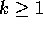bits can represent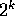values, show that k+1 bits can represent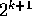values.

k+1 bit numbers can be broken into 2 groups:

• `0<k bits>`
• `1<k bits>`

Counting and using the inductive hypothesis, there are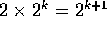k+1 bit numbers. QED.

Formulas for ranges of n-bit numbers?

## Floating Point Types

Memory allocation:

float, double, long double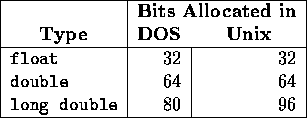Precision:

• float: 6 decimal digits
• double, long double: 15 digits

Range:

• float: 1.17E-38--3.4E+38
• double, long double: 2.22E-308--1.79E+308

# Common Runtime and Design Errors

• Division by 0
• Integer division
• Arithmetic overflow
• Integer: ``Wraparound''

Consider 4 bit signed/unsigned numbers

• Floating point: NaN
• Arithmetic underflow
• Representational errors
• Cancellation errors:
```#include <iostream.h>

int main()
{
double little = 1.0E-10;
double middle = 1.0E4;
double big = 1.0E6;
double bigger = 1.0E7;

cout << (middle + little) - middle << endl;
cout << (big + little) - big << endl;
cout << (bigger + little) - bigger << endl;

return 0;
}
```
Output:
```1.00044e-10
1.16415e-10
0
```

# Type Conversions

Expression types:

• Assignment expression --- type of the left operand; right hand value implicitly cast if need be
• All other expressions --- largest of int and the types of the two operands. Operator is resolved to this type

Relative ``sizes'' of types:

1. signed int
2. unsigned int
3. signed long int
4. unsigned long int
5. float
6. double
7. long double

Note: range increasing

Assignment conversion to ``smaller'' type unsafe (?)

Given the declarations, determines the expression types and the ``safeness:''

```int a;
unsigned int b;
char c;
float x;
double y;
long double z;

c = a % b - 5;
x = c - b * a;
b = c * c + b * a / 3;
a = y - c * a % b;
x = y * z;
c = +x * -y + 7 / z;
x = ((x - 6) * (x - 6) + y * y) / (z * z);
x += c + z;
```

Thomas P. Kelliher
Thu Feb 29 14:11:43 EST 1996
Tom Kelliher